# Outdoor Weather Monitoring System

This project will demonstrate the real prototype of smart cities product including sensor data manipulation using Google Data Studio.

IntermediateFull instructions provided15 hours2,572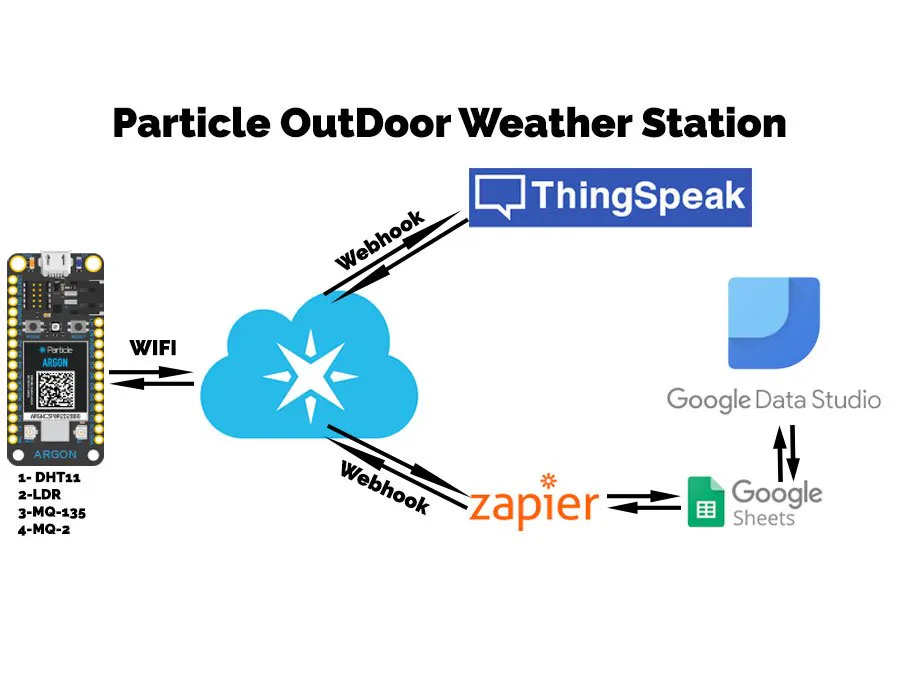## Things used in this project

### Hardware components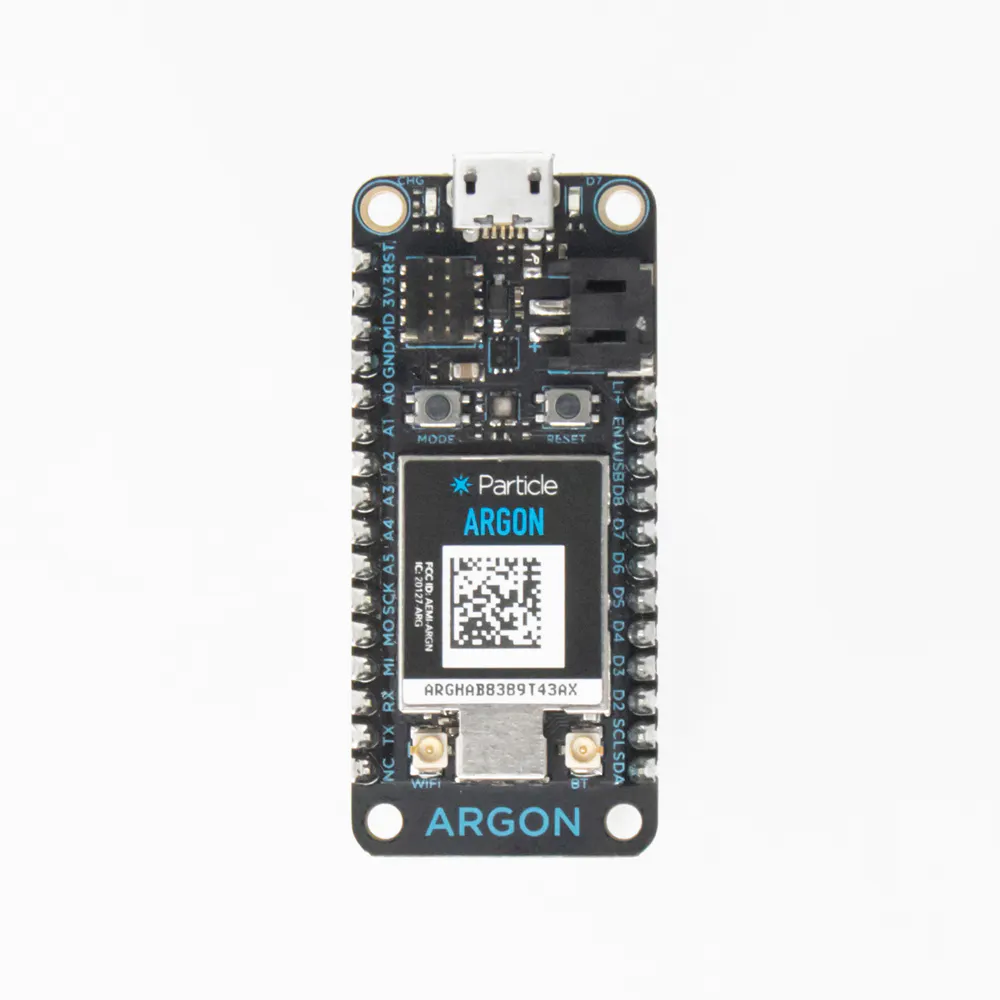Particle Argon
×1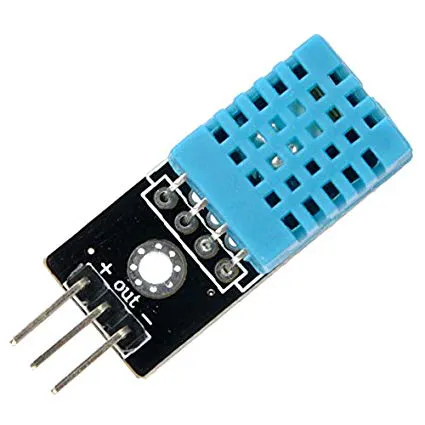DHT11 Temperature & Humidity Sensor (3 pins)
×1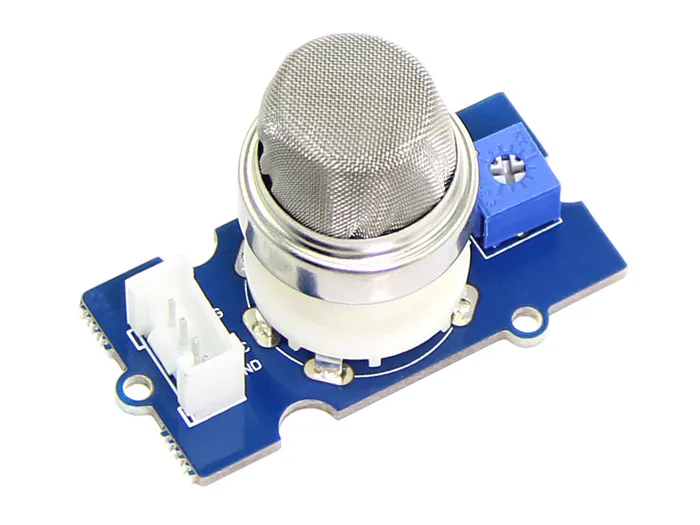Seeed Studio Grove - Gas Sensor(MQ2)
×1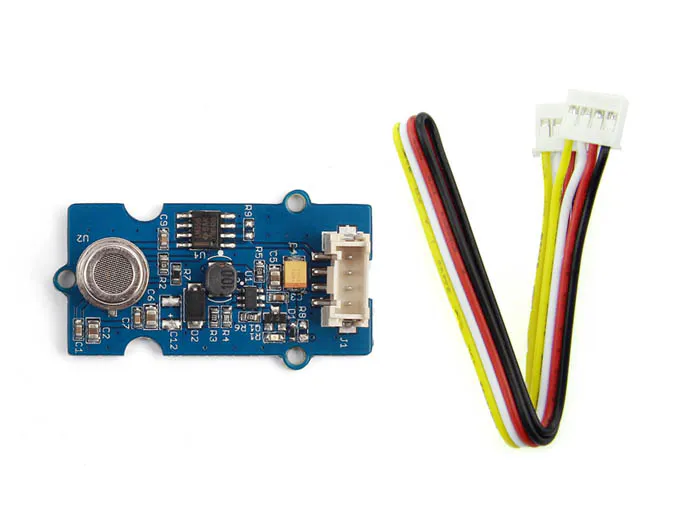Seeed Studio Grove - Air quality sensor v1.3
×1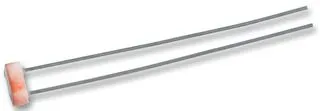LDR, 5 Mohm
×1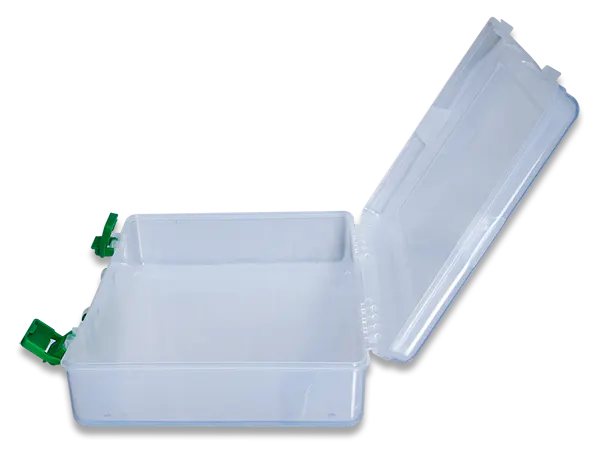Digilent Project Box: Jumbo-sized
×1
 18650 Lithim Ion Cell
×2
 Mini Solar Platte
×1

### Software apps and online servicesParticle Build Web IDEThingSpeak API
 Zapier## Schematics

### Design File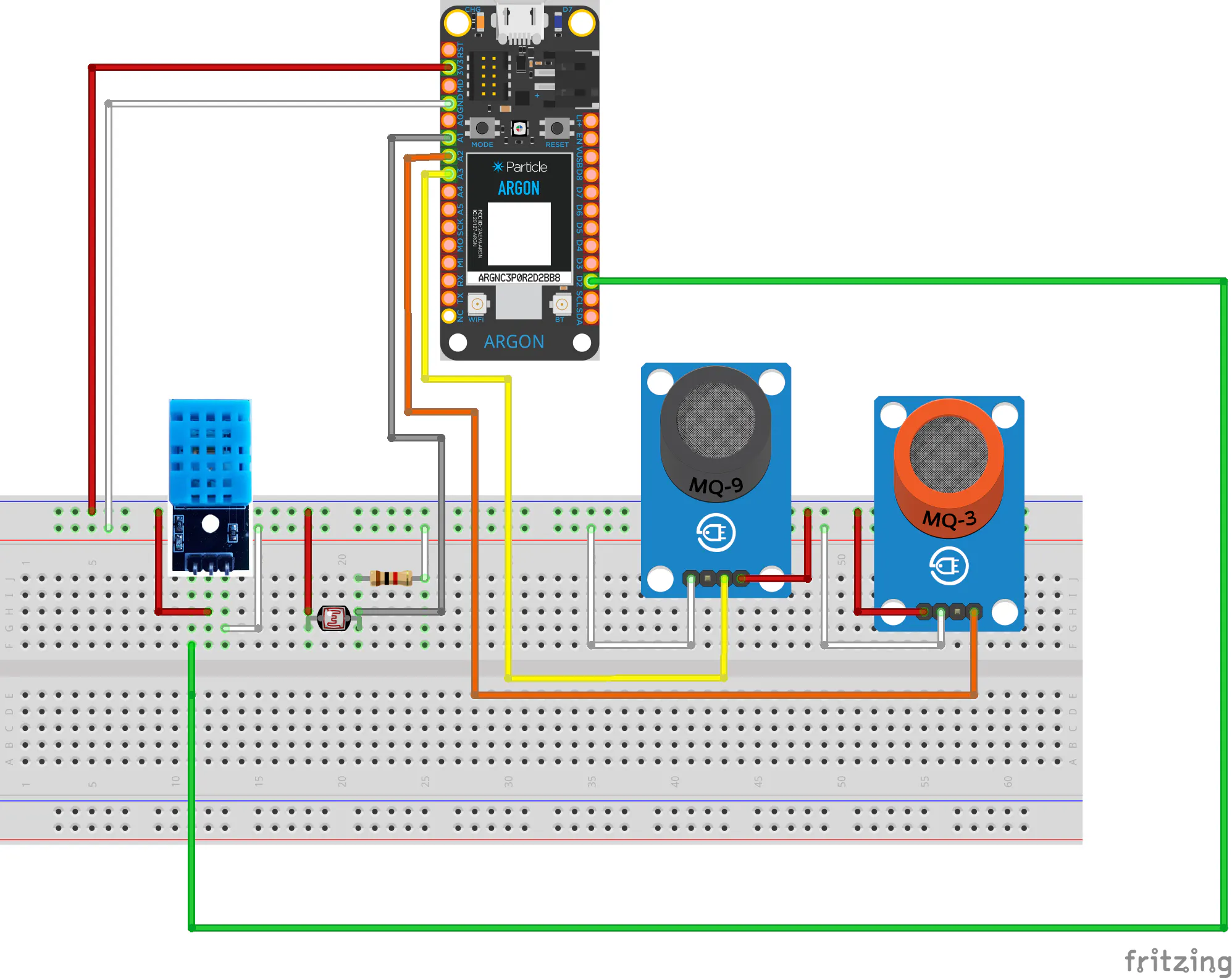## Code

### Weather Station Main File

Arduino
```/*
*   Outdoor Weather Montiring System
*   hackster.io/mafzal
*/

#define DHTPIN 2     // what pin we're connected to

// Uncomment whatever type you're using!
#define DHTTYPE DHT11		// DHT 11

DHT dht(DHTPIN, DHTTYPE);

SYSTEM_MODE(AUTOMATIC);

void setup() {
pinMode(A0,INPUT); //LDR PIN
pinMode(A1,INPUT); //MQ2 PIN
pinMode(A2,INPUT); //MQ135 PIN
Serial.begin(115200);
dht.begin();
}

void loop() {

// Wait a few seconds between measurements.
delay(2000);
// very slow sensor)
float h = dht.getHumidity();
float t = dht.getTempCelcius();

//Get Light Level

// Check if any reads failed and exit early (to try again).
// if (isnan(h) || isnan(t) || isnan(f)) {
if (isnan(h)) {
Serial.println("Failed to read from DHT sensor!");
h=t=0;
}

//Convert the Sensor Data into JSON Response For  Webhooks which will send the data to ThingSpeak & Zapier for further process

String Data="{\"field1\":\""+String(t)+"\","; //Temp
Data+="\"field2\":\""+String(h)+"\","; //Humdity
Data+="\"field3\":\""+String(LDR)+"\","; //LDR
Data+="\"field4\":\""+String(MQ135)+"\","; //MQ135
Data+="\"field5\":\""+String(MQ2)+"\"}"; //MQ2

Particle.publish("WeatherData", Data, PUBLIC);

Serial.print("Humid: ");
Serial.print(h);
Serial.print("% - ");
Serial.print("Temp: ");
Serial.print(t);
Serial.println("*C");
Serial.print("LDR: ");
Serial.println(LDR);
Serial.print("MQ2: ");
Serial.println(MQ2);
Serial.print("MQ135: ");
Serial.println(MQ135);
Serial.println(Time.timeStr());
// Wait a few 30 seconds between measurements.
delay(300000);
}
```

C/C++
```/* DHT library
*
* modified for Spark Core by RussGrue
* */

DHT::DHT(uint8_t pin, uint8_t type, uint8_t count) {
_pin = pin;
_type = type;
_count = count;
}

void DHT::begin(void) {
// set up the pins!
pinMode(_pin, INPUT);
pinSetFast(_pin);
}

float f;

switch (_type) {
case DHT11:
f = data;
return f;
case DHT22:
case DHT21:
f = data & 0x7F;
f *= 256;
f += data;
f /= 10;
if (data & 0x80)
f *= -1;
return f;
}
}
return NAN;
}

float DHT::getHumidity() {
}

float DHT::getTempCelcius() {
}

float DHT::getTempFarenheit() {
}

float DHT::getTempKelvin() {
}

float DHT::getHeatIndex() {
}

float DHT::getDewPoint() {
}

float DHT::convertFtoC(float f) {
return (f - 32) * 5 / 9;
}

float DHT::convertCtoF(float c) {
return c * 9 / 5 + 32;
}

float DHT::convertCtoK(float c) {
return c + 273.15;
}

float f;

switch (_type) {
case DHT11:
f = data;
return f;
case DHT22:
case DHT21:
f = data;
f *= 256;
f += data;
f /= 10;
return f;
}
}
return NAN;
}

float DHT::computeHeatIndex(float tempFahrenheit, float percentHumidity) {
// Wikipedia: http://en.wikipedia.org/wiki/Heat_index
return -42.379 +
2.04901523 * tempFahrenheit +
10.14333127 * percentHumidity +
-0.22475541 * tempFahrenheit * percentHumidity +
-0.00683783 * pow(tempFahrenheit, 2) +
-0.05481717 * pow(percentHumidity, 2) +
0.00122874 * pow(tempFahrenheit, 2) * percentHumidity +
0.00085282 * tempFahrenheit * pow(percentHumidity, 2) +
-0.00000199 * pow(tempFahrenheit, 2) * pow(percentHumidity, 2);
}

float DHT::computeDewPoint(float tempCelcius, float percentHumidity) {
double a = 17.271;
double b = 237.7;
double tC = (a * (float) tempCelcius) / (b + (float) tempCelcius) + log( (float) percentHumidity / 100);
double Td = (b * tC) / (a - tC);
return Td;
}

uint8_t laststate = HIGH;
uint8_t counter = 0;
uint8_t j = 0, i;
unsigned long currenttime;

// Check if sensor was read less than two seconds ago and return early
currenttime = millis();
// ie there was a rollover
}
return true; // return last correct measurement
//		delay(2000 - (currenttime - _lastreadtime));
}
/*
Serial.print("Currtime: "); Serial.print(currenttime);
*/

data = data = data = data = data = 0;

// pull the pin high and wait 250 milliseconds
pinSetFast(_pin);
delay(250);

// now pull it low for ~20 milliseconds
pinMode(_pin, OUTPUT);
pinResetFast(_pin);
delay(20);
noInterrupts();
pinSetFast(_pin);
delayMicroseconds(40);
pinMode(_pin, INPUT);

for ( i=0; i< MAXTIMINGS; i++) {
counter = 0;
counter++;
delayMicroseconds(1);
if (counter == 255) {
break;
}
}

if (counter == 255) break;

// ignore first 3 transitions
if ((i >= 4) && (i%2 == 0)) {
// shove each bit into the storage bytes
data[j/8] <<= 1;
if (counter > _count)
data[j/8] |= 1;
j++;
}
}

interrupts();

/*
Serial.println(j, DEC);
Serial.print(data, HEX); Serial.print(", ");
Serial.print(data, HEX); Serial.print(", ");
Serial.print(data, HEX); Serial.print(", ");
Serial.print(data, HEX); Serial.print(", ");
Serial.print(data, HEX); Serial.print(" =? ");
Serial.println(data + data + data + data, HEX);
*/

// check we read 40 bits and that the checksum matches
if ((j >= 40) &&
(data == ((data + data + data + data) & 0xFF)) ) {
return true;
}

return false;

}
```

C/C++
```/* DHT library
*
* modified for Spark Core by RussGrue
* */

#ifndef DHT_H
#define DHT_H

#include "application.h"
#include "math.h"

// how many timing transitions we need to keep track of. 2 * number bits + extra
#define MAXTIMINGS 85

#define DHT11 11
#define DHT22 22
#define DHT21 21
#define AM2301 21

class DHT {
private:
uint8_t data;
uint8_t _pin, _type, _count;
float convertFtoC(float);
float convertCtoF(float);
float convertCtoK(float);
float computeHeatIndex(float tempFahrenheit, float percentHumidity);
float computeDewPoint(float tempCelcius, float percentHumidity);

public:
DHT(uint8_t pin, uint8_t type, uint8_t count=6);
void begin(void);
float getHumidity();
float getTempCelcius();
float getTempFarenheit();
float getTempKelvin();
float getHeatIndex();
float getDewPoint();

};
#endif
```